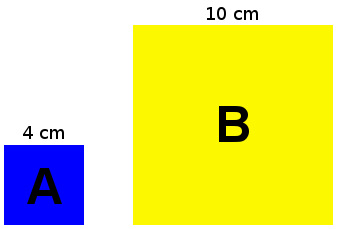# Scale Factor Calculator

 Length A mm cm meters km inches feet yards miles Length B mm cm meters km inches feet yards miles Scale Factor :
Your browser does not support the canvas element.

If you want to know what is the length under conversion at different scales, try this,scale length conversion tool, it helps us calculate the length quickly.

This calculator help us find the scale factor between two lengths, simply enter two lengths, it will automatically calculate the scale factor, supports different length units (mm, cm, m, km, in, ft, yd, mi), in addition corresponding visual graphic and formula, easy understanding the calculation process and the result.

### How to use the scale factor calculator

1. Enter the length of A and B
2. Number accept decimal or fraction, eg. 6, 12, 4.7, 1/2, 5 3/8
3. If the length units are different, choose the right unit
4. The result(scale factor) will be calculated automatically.

### How to figure out scale factor ?

In two similar geometric figures, the scale factor is the ratio of their corresponding sides, dividing the two corresponding lengths of the sides will gives the ratio, for example

#### What is the scale factor between 4 cm and 10 cm ?4 and 10 are divisible by 2
Length A : 4 ÷ 2 = 2
Length B : 10 ÷ 2 = 5
so the scale factor from A to B is 2:5

#### If 12 inches equals 3 inches, what is the scale factor ?

12 and 3 are divisible by 3
12 ÷ 3 = 4
3 ÷ 3 = 1
12:3 ratio simplified is 4:1
so the scale factor of 12 inches to 3 inches is 4:1

#### If 1/4 inches equals 2 feet, what is the scale factor ?

1⁄4 in = 1 ÷ 4 = 0.25 in
2 ft = 12 × 2 = 24 in
1 ÷ 0.25 = 4
24 × 4 = 96
0.25:24 ratio simplified is 1:96
so the scale factor of 1⁄4 inches to 2 feet is 1:96